# A Comprehensive Guide to Neural Machine Translation using Seq2Seq Modelling using PyTorch

September 13, 2020 • By BalakrishnaKumar

In this post, we will be building an LSTM based Seq2Seq model with the Encoder-Decoder architecture for machine translation without attention mechanism.

# 1. Introduction

Neural machine translation (NMT) is an approach to machine translation that uses an artificial neural network to predict the likelihood of a sequence of words, typically modeling entire sentences in a single integrated model.

It was one of the hardest problems for computers to translate from one language to another with a simple rule-based system because they were not able to capture the nuances involved in the process. Then shortly we were using statistical models but after the entry of deep learning the field is collectively called Neural Machine Translation and now it has achieved State-Of-The-Art results.

I want this post to be beginner-friendly, so a specific kind of architecture (Seq2Seq) showed a good sign of success, is what we are going to implement here.

So the Sequence to Sequence (seq2seq) model in this post uses an encoder-decoder architecture, which uses a type of RNN called LSTM (Long Short Term Memory), where the encoder neural network encodes the input language sequence into a single vector, also called as a Context Vector.

This Context Vector is said to contain the abstract representation of the input language sequence. This vector is then passed into the decoder neural network, which is used to output the corresponding output language translation sentence, one word at a time.

Here I am doing a German to English neural machine translation. But the same concept can be extended to other problems such as Named Entity Recognition (NER), Text Summarization, even other language models, etc.

# 2. Data Preparation and Pre-processing

For getting the data in the best way we want, I am using SpaCy (Vocabulary Building), TorchText (text Pre-processing) libraries, and multi30k dataset which contains the translation sequences for English, German and French languages

Torch text is a powerful library for making the text data ready for a variety of NLP tasks. It has all the tools to perform preprocessing on the textual data.

Let’s see some of the processes it can do,

1. Train/ Valid/ Test Split: partition your data into a specified train/ valid/ test set.
2. File Loading: load the text corpus of various formats (.txt,.json,.csv).
3. Tokenization: breaking sentences into a list of words.
4. Vocab: Generate a list of vocabulary from the text corpus.
5. Words to Integer Mapper: Map words into integer numbers for the entire corpus and vice versa.
6. Word Vector: Convert a word from a higher dimension to a lower dimension (Word Embedding).
7. Batching: Generate batches of the sample.

So once we get to understand what can be done in torch text, let’s talk about how it can be implemented in the torch text module. Here we are going to make use of 3 classes under torch text.

1. Fields :This is a class under the torch text, where we specify how the preprocessing should be done on our data corpus.
2. TabularDataset :Using this class, we can actually define the Dataset of columns stored in CSV, TSV, or JSON format and also map them into integers.
3. BucketIterator :Using this class, we can perform padding our data for approximation and make batches with our data for model training.

Here our source language (SRC — Input) is German and the target language (TRG — Output) is English. We also add 2 extra tokens “start of sequence” and “end of sequence” for effective model training.

After setting the language pre-processing criteria, the next step is to create batches of training, testing, and validation data using iterators.

Creating batches is an exhaustive process, luckily we can make use of TorchText’s iterator library.

Here we are using BucketIterator for effective padding of source and target sentences. We can access the source (german) batch of data using the .src attribute and it's corresponding (English) batch of data using the .trg attribute. Also, we can see the data before tokenizing it.

I just experimented with a batch size of 32 and a sample batch is shown below. The sentences are tokenized into a list of words and indexed according to the vocabulary. The “pad” token gets an index of 1.

Each column corresponds to a sentence, indexed into numbers and we have 32 such sentences in a single target batch and the number of rows corresponds to the maximum length of that sentence. Short sentences are padded with 1's to compensate for the length.

The table below (Idx.csv) contains the numerical indices of the batch, which is later fed into the word embedding and converted into dense representation for Seq2Seq processing.

The table below (Words.csv) contains the corresponding words mapped with the numerical indices of the batch.

# 3. Long Short Term Memory (LSTM)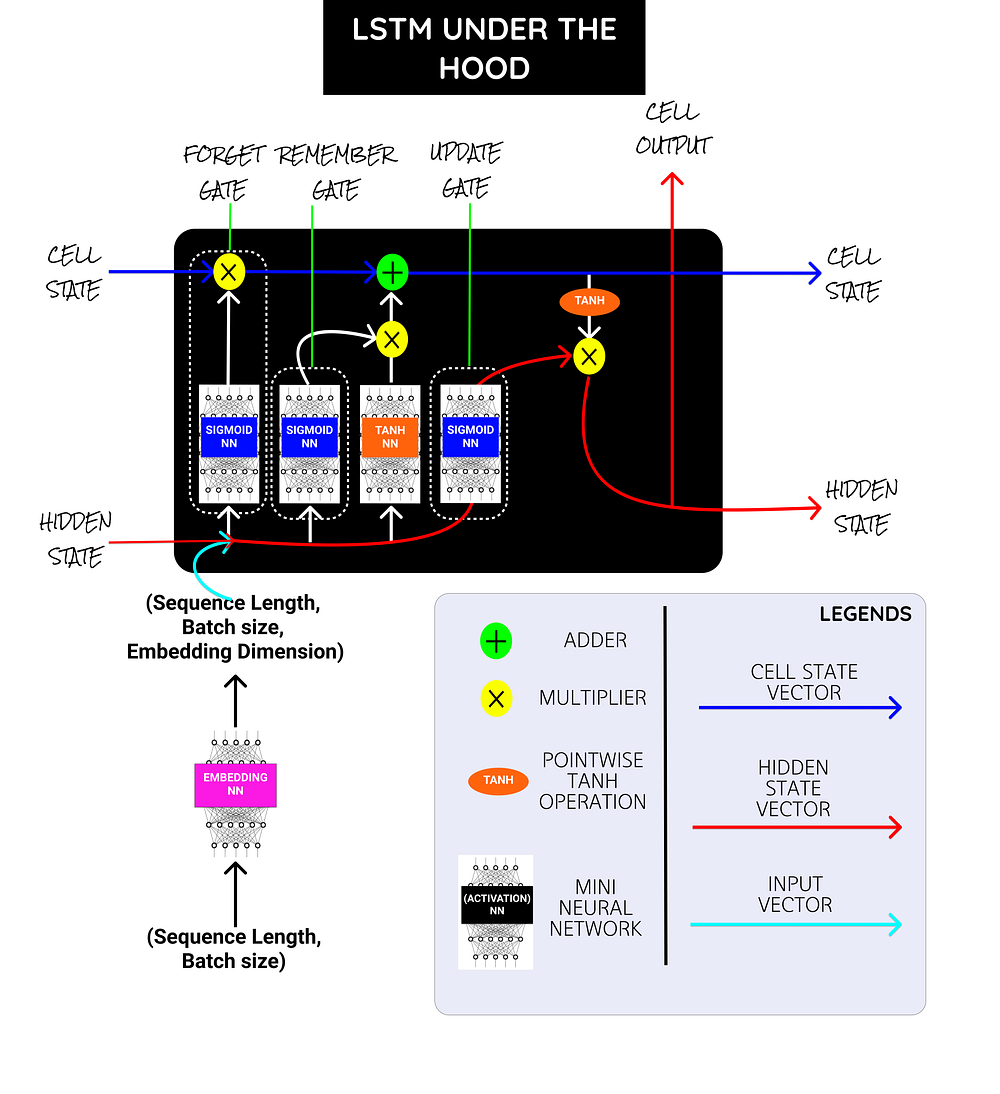LSTM - Under the hood

The above picture shows the units present under a single LSTM Cell. I will add some references to learn more about LSTM in the last and why it works well for long sequences.

But to simply put, Vanilla RNN, Gated Recurrent Unit (GRU) is not able to capture the long term dependencies due to its nature of design and suffers heavily by the Vanishing Gradient problem, which makes the rate of change in weights and bias values negligible, resulting in poor generalization.

Inside the LSTM cell, we have a bunch of mini neural networks with sigmoid and TanH activations at the final layer and few vector adder, Concat, multiplications operations.

Sigmoid NN → Squishes the values between 0 and 1. Say a value closer to 0 means to forget and a value closer to 1 means to remember.

Embedding NN → Converts the input word indices into word embedding.

TanH NN → Squishes the values between -1 and 1. Helps to regulate the vector values from either getting exploded to the maximum or shrank to the minimum.

## Gates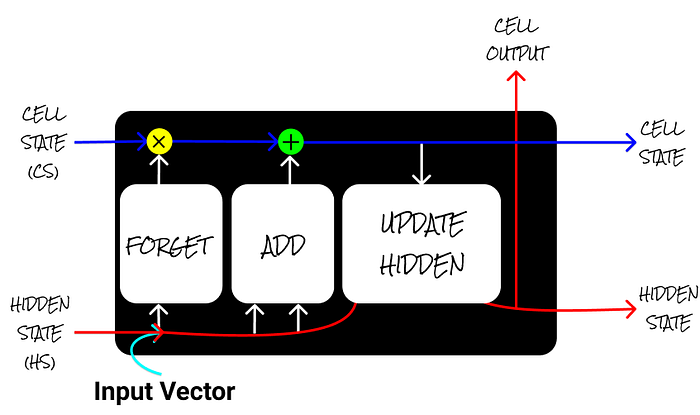LSTM - Gates

But LSTM has some special units called gates (Remember (Add) gate, Forget gate, Update gate), which helps to overcome the problems stated before.

Forget Gate → Has sigmoid activation in it and range of values between (0–1) and it is multiplied over the cell state to forget some elements. (“Vector” * 0 = 0)

Add Gate → Has TanH activation in it and range of values between (-1 to +1) and it is added over the cell state to remember some elements. (“Vector” * 1= “Vector”)

Update Hidden → Updates the Hidden State based on the Cell State.

The hidden state and the cell state are referred to here as the context vector, which are the outputs from the LSTM cell. The input is the sentence’s numerical indexes fed into the embedding NN.

# 4. Encoder Model Architecture (Seq2Seq)

Before moving to build the seq2seq model, we need to create an Encoder, Decoder, and create an interface between them in the seq2seq model.

Let’s pass the german input sequence “Ich Liebe Tief Lernen” which translates to “I love deep learning” in English.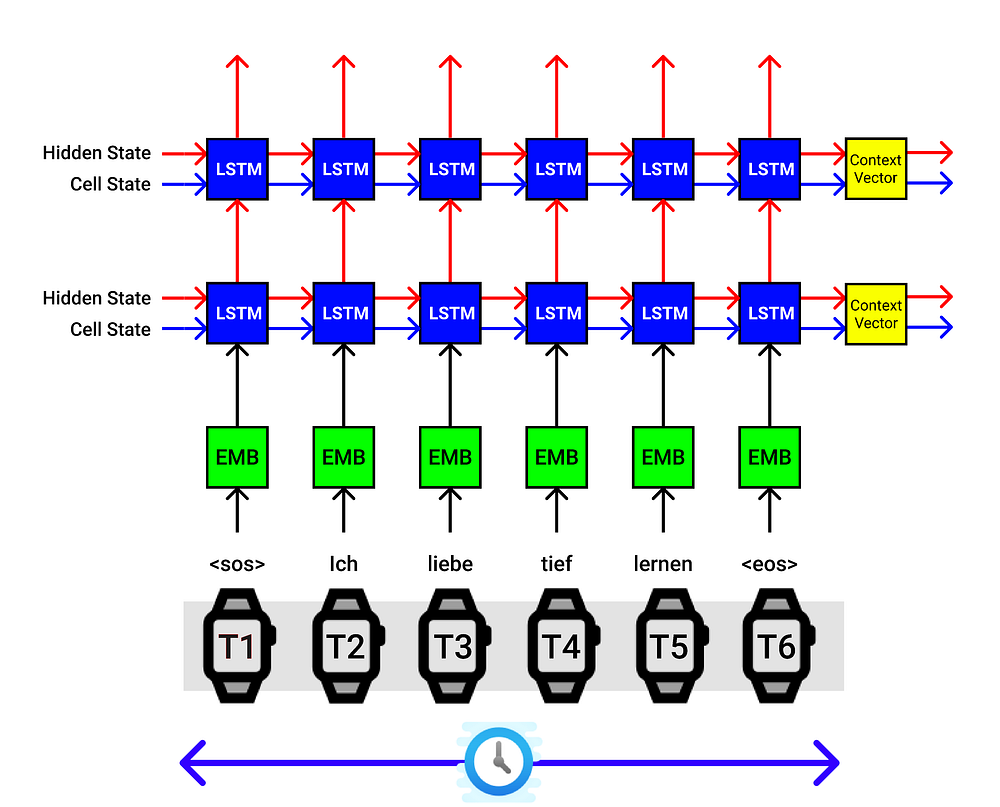LSTM Encoder Architecture. The X-axis corresponds to time steps and the Y-axis corresponds to batch size

For a lighter note, let’s explain the process happening in the above image. The Encoder of the Seq2Seq model takes one input at a time. Our input German word sequence is “ich Liebe Tief Lernen”.

Also, we append the start of sequence “SOS” and the end of sentence “EOS” tokens in the starting and in the ending of the input sentence.

Therefore at

1. At time step-0, the ”SOS” token is sent,
2. At time step-1 the token “ich” is sent,
3. At time step-2 the token “Liebe” is sent,
4. At time step-3 the token “Tief” is sent,
5. At time step-4 the token “Lernen” is sent,
6. At time step-4 the token “EOS” is sent.

And the first block in the Encoder architecture is the word embedding layer [shown in green block], which converts the input indexed word into a dense vector representation called word embedding (sizes — 100/200/300).

Then our word embedding vector is sent to the LSTM cell, where it is combined with the hidden state (hs), and the cell state (cs) of the previous time step and the encoder block outputs a new hs and a cs which is passed to the next LSTM cell. It is understood that the hs and cs captured some vector representation of the sentence so far.

At time step-0, the hidden state and cell state are either initialized fully of zeros or random numbers.

Then after we sent pass all our input German word sequence, a context vector [shown in yellow block] (hs, cs) is finally obtained, which is a dense representation of the word sequence and can be sent to the decoder’s first LSTM (hs, cs) for corresponding English translation.

In the above figure, we use 2 layer LSTM architecture, where we connect the first LSTM to the second LSTM and we then we obtain 2 context vectors stacked on top as the final output. This is purely experimental, you can manipulate it.

It is a must that we design identical encoder and decoder blocks in the seq2seq model.

The above visualization is applicable for a single sentence from a batch.

Say we have a batch size of 5 (Experimental), then we pass 5 sentences with one word at a time to the Encoder, which looks like the below figure.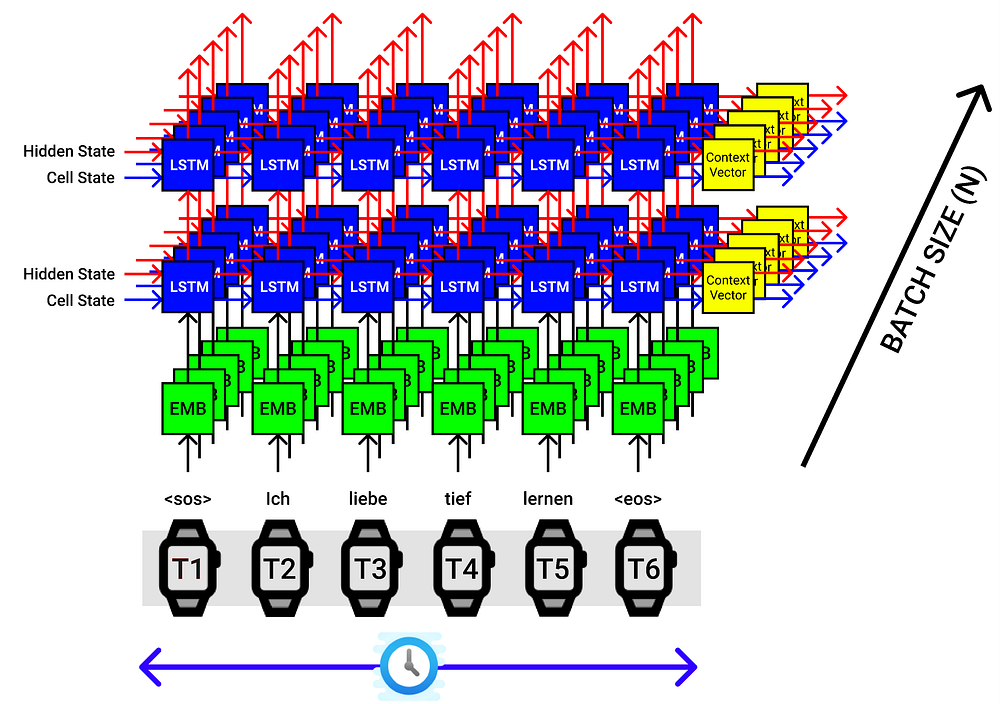LSTM Encoder for Batch Size of 5. The X-axis corresponds to time steps and the Y-axis corresponds to batch size.

# 6. Decoder Model Architecture (Seq2Seq)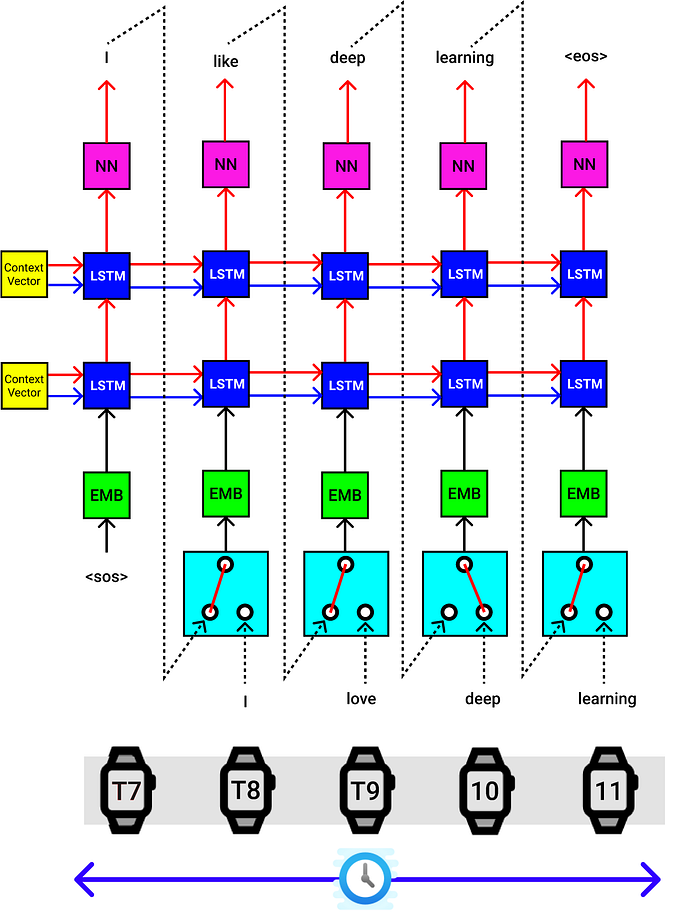LSTM Decoder Architecture. The X-axis corresponds to time steps and the Y-axis corresponds to batch size.

The decoder also does a single step at a time.

The Context Vector from the Encoder block is provided as the hidden state (hs) and cell state (cs) for the decoder’s first LSTM block.

The start of sentence “SOS” token is passed to the embedding NN, then passed to the first LSTM cell of the decoder, and finally, it is passed through a linear layer [Shown in Pink color], which provides an output English token prediction probabilities (4556 Probabilities) [4556 — as in the total vocabulary size of English language], hidden state (hs), Cell State (cs).

The output word with the highest probability out of 4556 values is chosen, hidden state (hs), and Cell State (cs) is passed as the inputs to the next LSTM cell and this process is executed until it reaches the end of sentences “EOS”.

The subsequent layers will use the hidden and cell state from the previous time steps.

## Teach Force Ratio:

In addition to other blocks, you will also see the block shown below in the Decoder of the Seq2Seq architecture. While model training, we send the inputs (German Sequence) and targets (English Sequence). After the context vector is obtained from the Encoder, we send them Vector and the target to the Decoder for translation.

But during model Inference, the target is generated from the decoder based on the generalization of the training data. So the output predicted words are sent as the next input word to the decoder until a token is obtained.

So in model training itself, we can use the teach force ratio (tfr), where we can actually control the flow of input words to the decoder.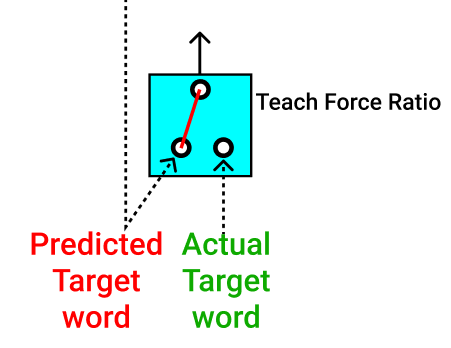TFR.

1. We can send the actual target words to the decoder part while training (Shown in Green Color).

2.We can also send the predicted target word, as the input to the decoder (Shown in Red Color).

Sending either of the word (actual target word or predicted target word) can be regulated with a probability of 50%, so at any time step, one of them is passed during the training.

This method acts like a Regularization. So that the model trains efficiently and fastly during the process.

The above visualization is applicable for a single sentence from a batch. Say we have a batch size of 4(Experimental), then we pass 4 sentences at a time to the Encoder, which provides 4 sets of Context Vectors, and they all are passed into the Decoder, which looks like the below figure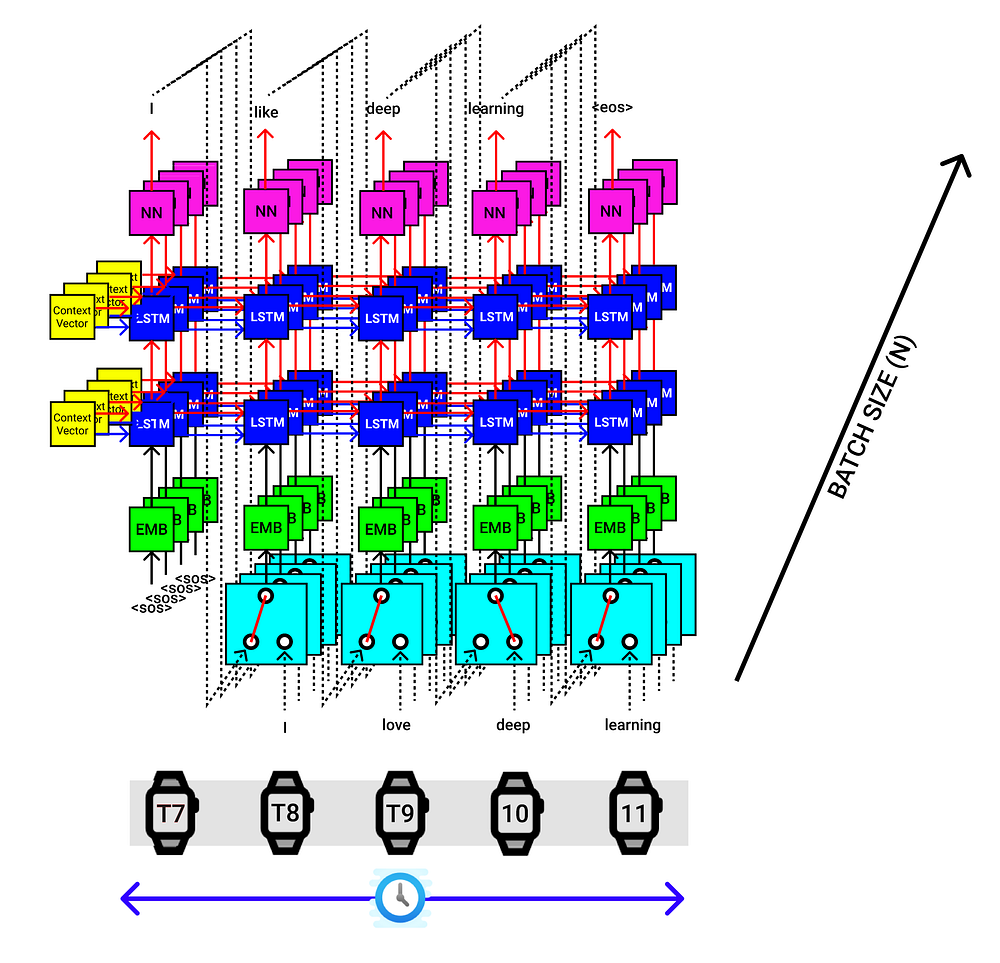LSTM Decoder for Batch Size of 4. The X-axis corresponds to time steps and the Y-axis corresponds to batch size.

# 8. Seq2Seq (Encoder + Decoder) Interface

The final seq2seq implementation for a single input sentence looks like the figure below.

1. Provide both input (German) and output (English) sentences.

2. Pass the input sequence to the encoder and extract context vectors.

3. Pass the output sequence to the Decoder, context vectors from the Encoder to produce the predicted output sequence.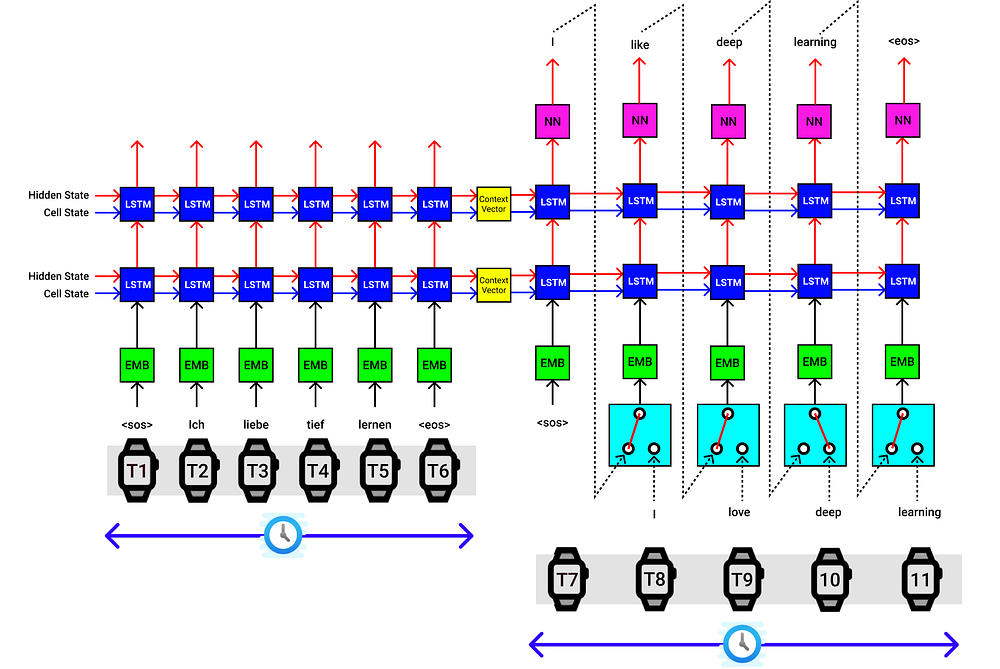Seq2Seq data flow diagram for a single sentence

The above visualization is applicable for a single sentence from a batch. Say we have a batch size of 4 (Experimental), then we pass 4 sentences at a time to the Encoder, which provide 4 sets of Context Vectors, and they all are passed into the Decoder, which looks like the below figure.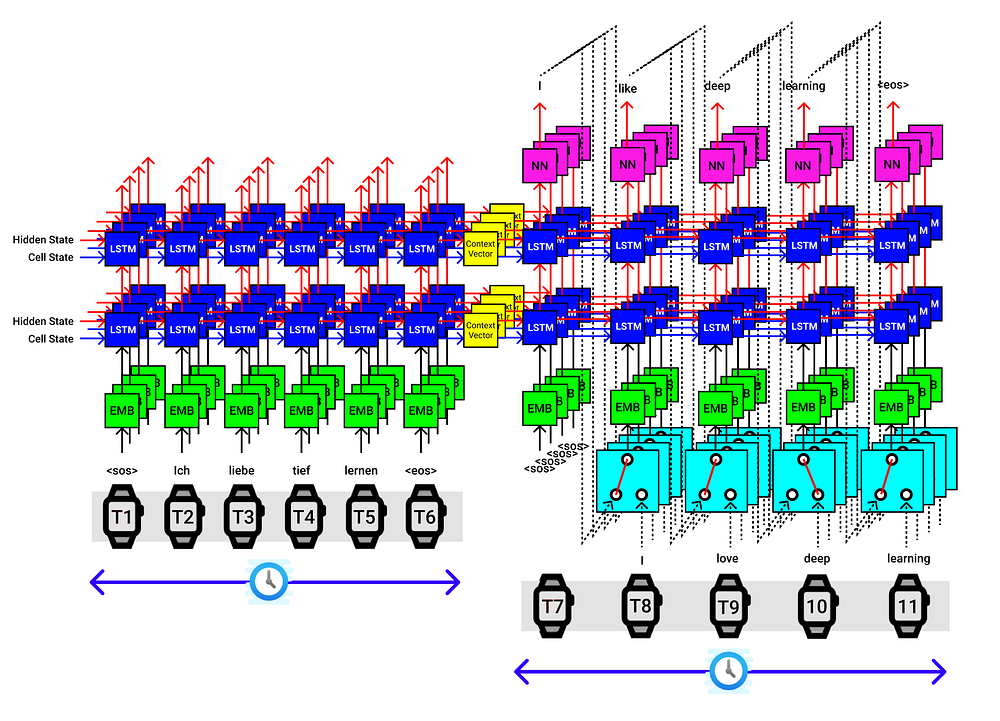Seq2Seq for a batch size of 4

# 10. Seq2Seq Model Training

## Training Loss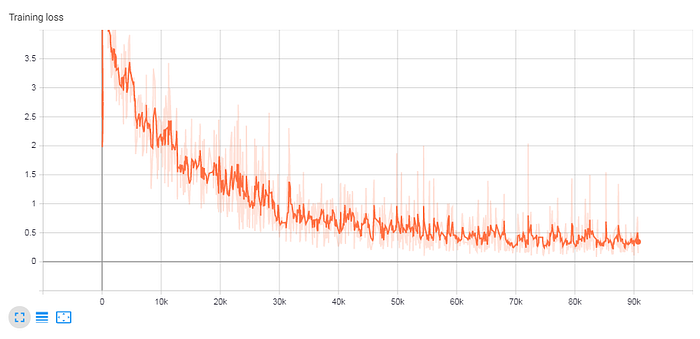The training loss shows a good trend.

# 11. Seq2Seq Model Inference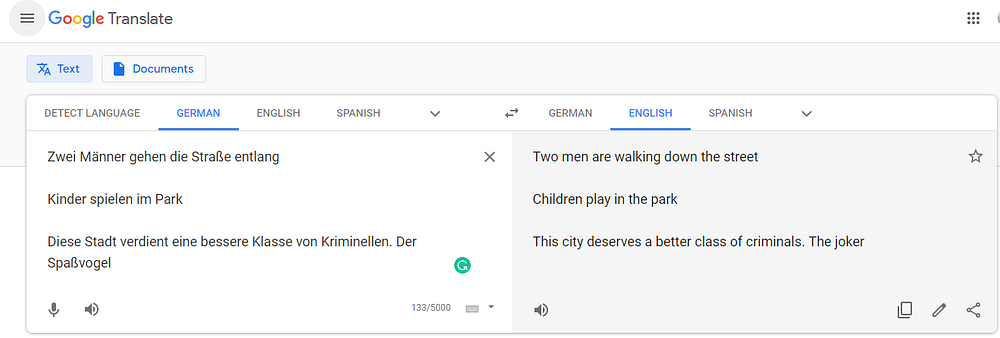Model Inference Samples.

Not bad, but clearly the model is not able to comprehend complex sentences. So in the upcoming series of posts, I will be enhancing the above model’s performance by altering the model’s architecture, like using Bi-directional LSTM, adding attention mechanism, or replacing LSTM with the Transformers model to overcome these apparent shortcomings.

# 12. Resources & References

I hope I was able to provide some visual understanding of how the Seq2Seq model processes the data, let me know your thoughts in the comment section.

Check out the Notebooks that contains the entire code implementation and feel free to break it.

Complete Code Implementation is available at,

References : LSTM, WORD EMBEDDING, DEEP LEARNING MODEL DEPLOYMENT ON AWS

Until then, see you next time.